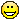# How to export an animation for a numerically solved system

Posted 10 years ago
7155 Views
|
6 Replies
|
7 Total Likes
|
 I've been trying to put together a double pendulum animation for my physics project. forces = {m1*       x1''[t] == (\[Lambda]1[t]/l1) x1[t] - (\[Lambda]2[t]/        l2) (x2[t] - x1[t]),     m1* y1''[t] == (\[Lambda]1[t]/l1) y1[t] - (\[Lambda]2[t]/        l2) (y2[t] - y1[t]) - m1* g,     m2* x2''[t] == (\[Lambda]2[t]/l2) (x2[t] - x1[t]),     m2* y2''[t] == (\[Lambda]2[t]/l2) (y2[t] - y1[t]) - m2* g}; constraints = {x1[t]^2 + y1[t]^2 ==      l1^2, (x2[t] - x1[t])^2 + (y2[t] - y1[t])^2 == l2^2};initialconditions = {x1 == 1, y1 == 0, x1' == 0, y1' == 0,   x2 == 1, y2 == -.6, x2' == 0,   y2' == 0}; constants = {g -> 9.8, m1 -> 1, m2 -> 1, l1 -> 1,   l2 -> .6}; solution = First[NDSolve[{forces, constraints, initialconditions} /.     constants, {x1, y1, x2, y2, \[Lambda]1, \[Lambda]2}, {t, 0, 100},    Method -> {"IndexReduction" -> {"Pantelides",        "ConstraintMethod" -> "Projection"}}]]; Animate[ Graphics[{{PointSize[.025], {Gray, Point[{x1[t], y1[t]}]}, {Orange,       Point[{x2[t], y2[t]}]},      Line[{{0, 0}, {x1[t], y1[t]}, {x2[t], y2[t]}}]} /.     solution, {Orange,     Line[Map[Function[Evaluate[{x2[#], y2[#]} /. solution]],       Range[0, t, .025]]]}, {Gray, Opacity[.5],     Line[Map[Function[Evaluate[{x1[#], y1[#]} /. solution]],       Range[0, t, .025]]]}}, PlotRange -> {{-1.6, 1.6}, {-1.8, 0}},   Axes -> False, Ticks -> False, ImageSize -> 500], {{t, , "Time"}, 0,   7, .0000001}, DefaultDuration -> 40, RefreshRate -> 30]I've been trying to export this using Export["m.avi",%]But the result I get is a 4 second long video that looks like this the entire timeThe output Mathematica gives after the animate command is the kind of thing i'm looking for -- a 40 second video of the double pendulum starting from the initial conditions of rest. Am I doing anything wrong?
6 Replies
Sort By:
Posted 10 years ago
 Lucas, this is a very lovely application and we decided to give its animated GIF below. You may also find it useful to check similar applications at the Wolfram Demonstration Project.Posted 10 years ago
 Lucas, it is a beautiful animation, but there is a typo in your posted code that causes the problem - as you can see on the image below you did not specify initial moment of time - it should be zero between those 2 comas. On a general note, the movie you export will be too fast. For precision control I suggest using Table, not animate and control the number of frames - table values - the more frames you have the slower is the movie. Basically this you need this approach, just change GIF to AVI: How to export GIFsPosted 10 years ago
 Sam, from my experience leaving a blank space within the manipulate value {{t,,"Time"},0,10}will leave the value as default. I just wanted to give the variable a name "Time."I modify the speed usingDefaultDuration -> 40Thanks for the GIF advice though, I think I'll do that!! It looks so nice, I worked hard getting it right!Posted 10 years ago
 These 2 versions will work as interfaces but 1st will fail on export. My guess is that automated procedure that computes total number of frames and duration need specified initial value to make a movie.Export["test.avi", Manipulate[t, {{t, , "Time"}, 0, 10}]]Export["test.avi", Manipulate[t, {{t, 0, "Time"}, 0, 10}]]
Posted 10 years ago
 Frank, you can easily upload animated GIFs to the community - as we did for your post. It is done in the same way you upload a regular image. To read how please refer to: How to type up a post: editor tutorial & general tips
Posted 10 years ago
 I generated an animated GIF in code that is here: Animating Optimization with the Gradient Projection Method